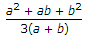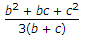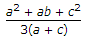# Civil Engineering - Applied Mechanics

### Exercise :: Applied Mechanics - Section 4

6.

The units of inertia of mass, are

 A. kg/m B. kg/m2 C. m4 D. m3 E. kg-m2.

Explanation:

No answer description available for this question. Let us discuss.

7.

A sphere is resting on two planes BA and BC which are inclined at 45° and 60° respectively with the horizontal. The reaction on the plane BA will be

 A. less than that on BC B. more than that of BC C. equal to that on BC D. zero E. none of these.

Explanation:

No answer description available for this question. Let us discuss.

8.

A projectile is fired at an angle θ to the vertical. Its horizontal range will be maximum when θ is

 A. 0° B. 30° C. 45° D. 60° E. 90°.

Explanation:

No answer description available for this question. Let us discuss.

9.

A load of 500 kg was lifted through a distance of 13 cm. by an effort of 25 kg which moved through a distance of 650 cm. The mechanical advantage of the lifting machine is

 A. 15 B. 18 C. 20 D. 26

Explanation:

No answer description available for this question. Let us discuss.

10.

The centre of gravity of a trapezoidal dam section whose top width is a, bottom width is b and the vertical side is a, from its vertical face is

 A.B.C.D. none of these.